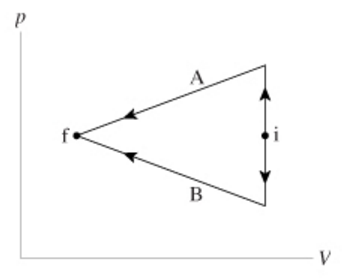# Problem: The figure (Figure 1) shows two different processes taking an ideal gas from state i to state f.Part A. Is the work done on the gas in process A greater than, less than, or equal to the work done in process B?A. WA  = WB B. WA  &gt; WB C. WA  &lt; WB

###### FREE Expert Solution

Work done on the gas:

$\overline{){\mathbf{W}}{\mathbf{=}}{\mathbf{\int }}{\mathbf{P}}{\mathbf{d}}{\mathbf{V}}}$

Since the initial and the final pressure are constant, this is an isobaric process.

This implies that ΔV is positive if the gas is expanding.

On the other hand, ΔV is negative if the gas compresses and the work done is positive.###### Problem Details

The figure (Figure 1) shows two different processes taking an ideal gas from state i to state f.Part A. Is the work done on the gas in process A greater than, less than, or equal to the work done in process B?

A. WA  = WB

B. WA  > WB

C. WA  < WB